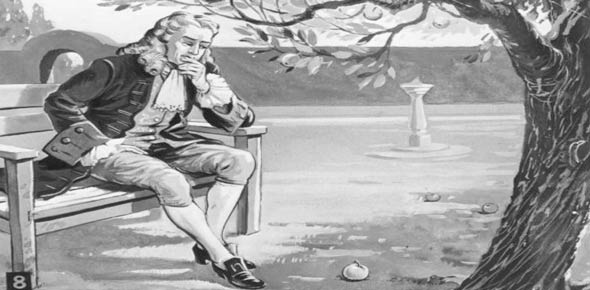# Newton's Laws

6 Questions | Total Attempts: 59SettingsWhat do you remember?

Related Topics
• 1.
How many Laws of Motion are there?
• A.

1

• B.

2

• C.

3

• D.

4

• 2.
If forces on an object are imbalanced it will
• A.

Stay in one place

• B.

Move upwards

• C.

Move in the direction of the greater force

• D.

Move in the direction of the smaller force

• 3.
A pencil on the table has many forces acting upon it. If gravity is pulling down what is also true?
• A.

The table is pushing up on the pencil

• B.

The table doesn't exert a force

• C.

The air keeps the pencil up

• D.

The pencil has no force of it's own

• 4.
If an car is traveling at 60mph it will maintain a constant speed as long as forces are balanced.
• A.

True

• B.

False

• 5.
If an object moves in one direction there has to be an equal opposite force.
• A.

True

• B.

False

• 6.
An object accelerates if...
• A.

There are opposite forces

• B.

Something changes

• C.

Forces are imbalanced

• D.

Greater force is applied